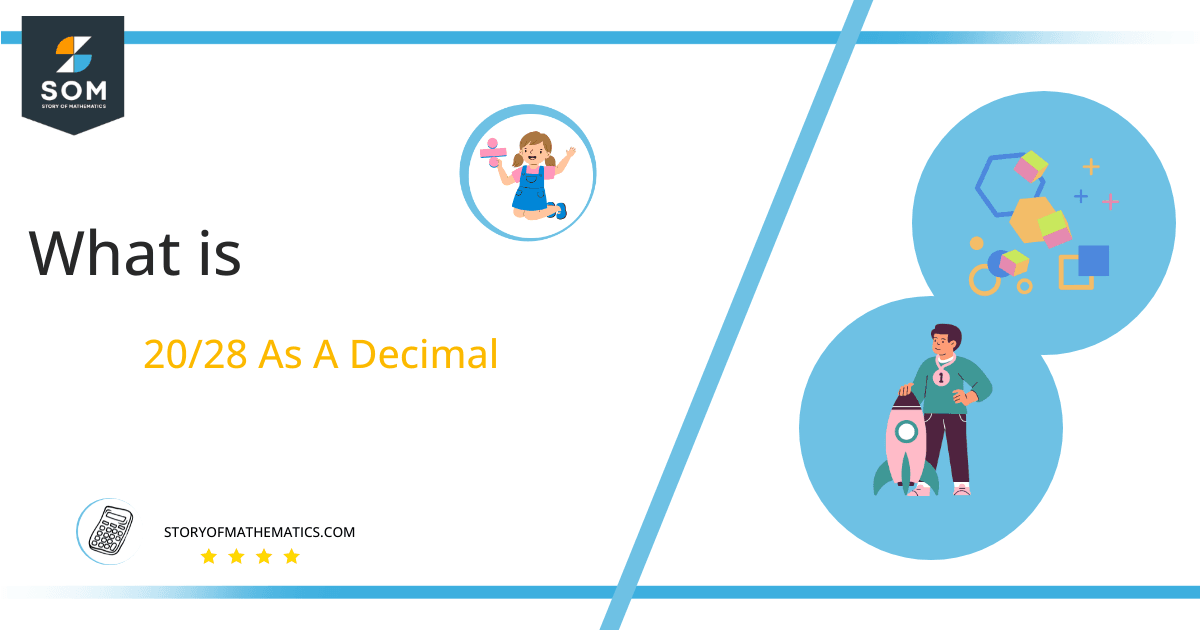# What Is 20/28 as a Decimal + Solution With Free Steps

The fraction 20/28 as a decimal is equal to 0.714.

Improper fractions are unique fractions that have their numerator value greater than the denominator value. These fractions are usually simplified into a mixed fraction form. They are greater than the value 1 and are converted into the decimal form using the Long division process

Here, we are more interested in the division types that result in a Decimal value, as this can be expressed as a Fraction. We see fractions as a way of showing two numbers having the operation of Division between them that result in a value that lies between two Integers.Now, we introduce the method used to solve said fraction to decimal conversion, called Long Division, which we will discuss in detail moving forward. So, let’s go through the Solution of fraction 20/28.

## Solution

First, we convert the fraction components, i.e., the numerator and the denominator, and transform them into the division constituents, i.e., the Dividend and the Divisor, respectively.

This can be done as follows:

Dividend = 20

Divisor = 28

Now, we introduce the most important quantity in our division process: the Quotient. The value represents the Solution to our division and can be expressed as having the following relationship with the Division constituents:

Quotient = Dividend $\div$ Divisor = 20 $\div$ 28

This is when we go through the Long Division solution to our problem. Given is the Long division process in Figure 1:Figure 1

## 20/28 Long Division Method

We start solving a problem using the Long Division Method by first taking apart the division’s components and comparing them. As we have 20 and 28, we can see how 20 is Smaller than 28, and to solve this division, we require that 20 be Bigger than 28.

This is done by multiplying the dividend by 10 and checking whether it is bigger than the divisor or not. If so, we calculate the Multiple of the divisor closest to the dividend and subtract it from the Dividend. This produces the Remainder, which we then use as the dividend later.

Now, we begin solving for our dividend 20, which after getting multiplied by 10 becomes 200.

We take this 200 and divide it by 28; this can be done as follows:

200 $\div$ 28 $\approx$ 7

Where:

28 x 7 = 196

This will lead to the generation of a Remainder equal to 200 – 196 = 4. Now this means we have to repeat the process by Converting the 4 into 40 and solving for that:

40 $\div$ 28 $\approx$ 1

Where:

28 x 1 = 28

This, therefore, produces another Remainder which is equal to 40 – 28 = 12. Now we must solve this problem to Third Decimal Place for accuracy, so we repeat the process with dividend 120.

120 $\div$ 28 $\approx$ 4

Where:

28 x 4 = 112

Finally, we have a Quotient generated after combining the three pieces of it as 0.714, with a Remainder equal to 8.Images/mathematical drawings are created with GeoGebra.